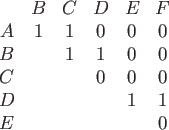Next: Non-trivial Monotone Graph Properties Up: Preliminaries Previous: Graph Properties:   Contents

#### Representing Graphs as Bit Strings:

Before we begin we need a way to represent a graph as a bit string, and graph properties as Boolean functions on that bit string if we wish to apply Theorem 2.1.1 or any of its derivatives. When representing graphs as bit strings it is helpful to restrict the types of graphs we will consider.

A simple graph has no multiple edges or loops. The adjacency matrix A of a simple directed graph has aij = 1 if and only if there is a directed edge from vertex i to vertex j. Since aii = 0 for all vertices i of a simple graph, we need only V(V - 1) bits to represent such graphs with V vertices. For undirected graphs, the adjacency matrix is symmetric across the diagonal. Thus we can represent any undirected simple graph with a bit string of length V(V - 1)/2. A simple undirected graph is depicted in Figure 3.1, its above-diagonal adjacency matrix representation can be seen in Table 3.1. Now that we can represent a graph by its above diagonal adjacency matrix, graph properties are simply Boolean functions on matrix representations.Next: Non-trivial Monotone Graph Properties Up: Preliminaries Previous: Graph Properties:   Contents
Matthew Hayward Lower Query Bounds in the Quantum Oracle Model GitHub Repository